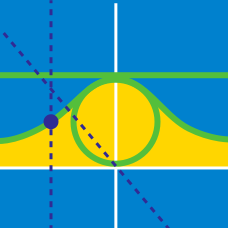Calculus

# Parametric Equations - Tangent

Consider the polar curve $r\theta = 6$. What is the slope of the tangent line of the polar curve at $\theta = \pi$?

Given the curve $x=1-2t, y=5-4t+2t^2,$ what is the equation of the tangent line to the curve at $x=5 ?$

For the curve given by $x=\frac{9t}{1+t^2}, y=\frac{1-t^2}{1+t^2},$ what is the equation of the tangent line to the curve for $t=2 ?$

Consider the polar curve $r = 22 \sin \theta$. What is the slope of the tangent line at $\theta = \frac{\pi}{6}$?

A curve is defined by $(x,y) =\left(\cos^3 \theta, \sin^3 \theta \right)$. If the equation for the tangent line at $\theta=\frac{2}{3}\pi$ is $y=ax+b$, what is the value of $a \times b$?

×

Problem Loading...

Note Loading...

Set Loading...